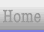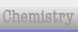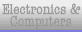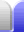# Simplifying

Simplying is combining like terms or cancelling common factors in fractions.

### Combining like terms

Terms are called "like terms" when they have the same unknowns (for example, x) to the same power. Their coefficients (the numbers in front of each term) can be the same or different.

The result of the example expansion on the expanding page has 8 terms, but only 5 different unknown and exponent combinations. We'll write the like terms next to each other then combine the like terms.

 48x2 + 24x3 + 12x4 + 6x5 - 24x3 - 12x4 - 6x5 - 3x6 = 48x2 + 24x3 - 24x3 + 12x4 - 12x4 + 6x5 - 6x5 - 3x6 = 48x2 + 0x3 + 0x4 + 0x5 - 3x6 = 48x2 - 3x6

And so we have our expanded and simplified answer.

3x2(4 + x2)(2 + x)(2 - x) = 48x2 - 3x6Home Astronomy Chemistry Electronics & Computers Mathematics Physics Field Trips Turn on javascript for email link